# Understanding Spectral Clustering

Some problems are linear, but some problems are non-linear. I presume that you started your education discussing and solving linear problems which is a natural starting point. For non-linear problems solutions often involve an initial processing step. The aim of that initial step is to transform the problem such that it has, again, linear flavor.

A textbook example is the logistic regression, a tried-and-true recipe for getting the best linear boundary between two classes. In a standard neural network model, you will find logistic regression (or multinomial regression for multi-class output) applied on transformed data. Few preceding layers are “devoted” to transform a non-separable input-space into something which linear methods could handle, allowing the logistic regression to solve the problem with relative ease.

The same rationale holds for spectral clustering. Rather than working with the original inputs, work first with a transformed data which would make it easier to solve, and then link back to your original inputs.

Spectral clustering is an important and up-and-coming variant of some fairly standard clustering algorithms. It is a powerful tool to have in your modern statistics tool cabinet. Spectral clustering includes a processing step to help solve non-linear problems, such that they could be solved with those linear algorithms we are so fond of. For example, the undeniably popular K-means.

Contents

### Motivation for spectral clustering

“Use a picture. It’s worth a thousand words” (Tess Flanders)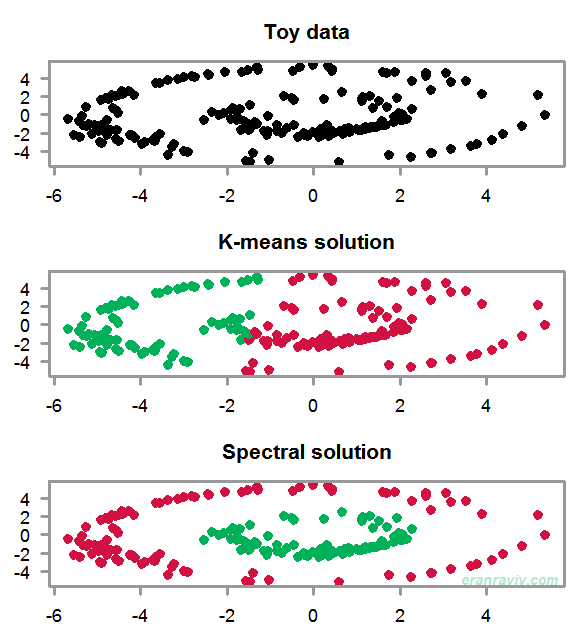The goal is to create two disjoint clusters. Our toy data has these “two rings” shape to it. You can see that because the data is not linearly separable, the K-means solution does not make much sense. We need to account for the particularly non-linear structure in the data. The way to do that One way to do that is to use the eigensubspace of the data, not even of the data actually, but of a similarity (affinity) matrix of the data. Bit obscure now, but some clarity is en route.

### A typical spectral clustering algorithm

Here is the formal algorithm. It is taken from a paper called “On Spectral Clustering” (see references). However, I complement each of the steps with my own explanation for what the hell is going on there. Deep breath, here we go.

1. Form the affinity matrixdefined by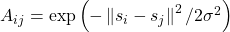ifand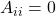. We compute the distance between each two points and collect those distances in a matrix. The diagonal is set to zero. The distance here is not something you may be used to, like euclidean for example. But look closely, it has some resemblance. Say you want to quantify similarity rather than distance. One way to do that is to use the inverse. For example, if the distance between two points is ‘tiny'(=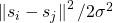), the points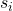andare similar and the quantity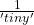is large, while if the distance between the two point is ‘large’ then similarity should be very small, and so taking the inverse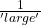would work for us as it would result in a small value. The ugly exponent expression does the same, only instead of taking the inverse we use another function which dictates a more aggressive decay than that of the inverse function; namely. This function is called Gaussian kerne and is widely used. Now that you understand the idea: moving from distance to similarity in that way, you don’t have to use a Gaussian kernel for your build. As long as you keep in line with the direction: large (small) distance –> small (large) similarity, you can choose some other function which conforms to this story. I have also seen people academics use the t-distribution rather than the Gaussian. In my opinion it needlessly complicates the math. I am doubtful about the added value from tweaking the decay rate around that kernel function, but at the same time perhaps I don’t know enough about this topic.
2. Defineto be the diagonal matrix whose-element is the sum of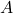‘s-th row, and construct the matrix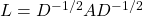. The matrix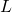is referred to by the somewhat bombastic name: graph Laplacian matrix, not sure where the name comes from. Now, what is the meaning of? In graph theory there is a notion of degree. A “too-big-to-fail” bank would have a high degree since a lot of other banks do business with it. In graph theory terms we denote the number of banks connected to that bankas the degree. Sinceis symmetric, you could think about either rows ofor the columns of. Say our aforementioned bank sits in row number i=4. We sum over all the entries in row, which would mean calculating the strength-of-contentedness of bankwith the other banks ()*. So that is. Becauseis a diagonal matrix,is a column-scaled and row-scaled version of(see the Appendix for the formal reason). The matrixis scaled with the strength-of-contentedness the rows and columns. Ifwould not be symmetrical then I guesswould be different for the rows and columns and it would go under the literature of directed graphs, as opposed to our undirected version here. For us it doesn’t matter if bankis indebted to bankor the other way around. How does the matrixrelates to our original data? Good one. The matrixsays something about the power of connectedness of each data point to other data points. The matrixsays nothing about the location of the point on the graph, rather it carries the graph-structure information; how crowded some data points around other data points, think local density. That is the main reason for the sensible solution presented in the figure’s bottom panel.
3. Find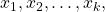thelargest eigenvectors of(chosen to be orthogonal to each other in the case of repeated eigenvalues), and form the matrix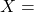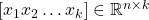by stacking the eigenvectors in columns. This step is just a conventional transformation of the matrix. Sincecontains graph-structure information, the “factors” that are created by the eigenvalue decomposition refers to areas of high-disconnectedness; simply put: where is the “action” in the graph? And the eigenvector could be thought of as the loadings on those factors. So if rowloads heavily on say the first two factors, it would mean that rowis important, in terms of what is going on around it. Remember we are still silent about the original data (we moved to distances, and from there to eigendecomposition), but we are getting there.
4. Form the matrixfromby renormalizing each of‘s rows to have unit length: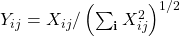. This step puts all rows on the same footing. Think about it as working with the correlation matrix rather than the covariance matrix.
5. Treating each row ofas a point in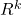, cluster them intoclusters via K-means or any other algorithm that attempts to minimize distortion. If you are looking for research ideas, not enough is known regarding the impact of using K-means versus variants thereof, or even completely different clustering algorithms, on the eventual clustering solution. For example, will hierarchical clustering delivers better clustering solutions, would regularization? See here for an R package to evaluate clustering solutions.
6. Finally(!), assign the original pointto clusterif and only if rowof the matrixwas assigned to cluster. This is where we link back to the original data input.

A brief recap of the sequence:

• compute Affinity matrixfrom our data –>
• transform using(apt notation for degree) –>
• apply eigen-decomposition on–>
• go back to the actual data which is denoted.

So by pre-processing and transforming our original data before applying our clustering algorithm we could get a better separation of classes. This is useful when the nature of the problem is non-linear. Before we adjourn, here you can find how to code it almost from scratch.

### Coding example

Generate fake rings data (code in the appendix for this), in the code it is the object dat. The following couple of lines simply generate the K-means solution and plot it.

Now for the spectral clustering solution:

* In our case the entries ofare not only 0’s and 1’s (connected or not), but numbers which quantify similarity.

### Appendix and references

Ng, Andrew Y., Michael I. Jordan, and Yair Weiss. “On spectral clustering: Analysis and an algorithm.” Advances in neural information processing systems. 2002.

A Tutorial on Spectral Clustering

Data Clustering: Theory, Algorithms, and ApplicationsTwo propositions
The following couple of proposition explain why whenis a diagonal matrix, then the matrixis a column-scaled and row-scaled version of:
Proposition: Let A be a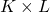matrix andbe adiagonal matrix. Then, the productis amatrix whose i-th row is equal to the i-th row of A multiplied by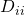(for every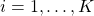).

Proposition: Let A be amatrix andbe adiagonal matrix. Then, the product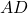is amatrix whose-th column is equal to the-th column of A multiplied by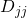(for every).
(source)

Code for the data